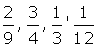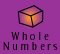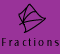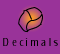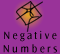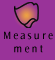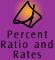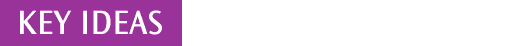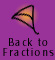Equivalent Fractions

Example 1: Folded paper model of equivalent fractions

Fractions which represent the same value are called equivalent fractions. We can see from the movie that 1/2, 2/4 and 4/8 all represent the same amount of piece of paper, therefore they are equivalent fractions. Each of these fractions represents the same number, it is just the way the number is named that changes.

Example 2: 2/3 = 8/12

 Setting Out Thinking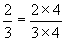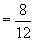How can thirds be changed into twelfths?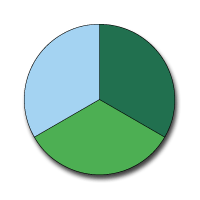We can divide each third into four equal parts.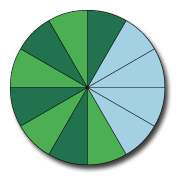There are 4 twelfths in each third, so there are 8 twelfths in 2/3. We have effectively multiplied the numerator and the denominator by 4 to get 8/12.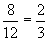To change 8/12 back to 2/3 we could divide both the numerator and the denominator by 4.

Example 3: 2/3 = 10/15

 Setting Out Thinking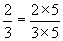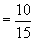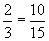How can thirds be changed into fifteenths? We know that 3 x 5 = 15, so we can divide each third into five equal parts. There are 5 fifteenths in each third, so there are 10 fifteenths in 2/3. We have effectively multiplied the numerator and the denominator by 5 to get 10/15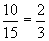To change 10/15 back to 2/3 we could divide both the numerator and the denominator by 5.

Recognising patterns

Equivalent fractions enable us to rename fractions so that we can compare, add and subtract them. We can generate equivalent fractions by recognising the patterns they form.

Example 4: What are the missing numbers in this sequence?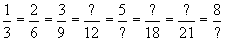....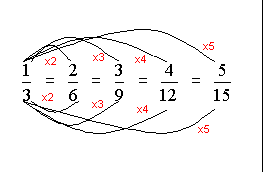To find each equivalent fraction in this sequence we have multiplied both the numerator and denominator of the original fraction, 1/3, by the same number for each fraction.

The fraction wall pictured below illustrates that 1/3 is the same as 2/6 which is the same as 3/9 and so on.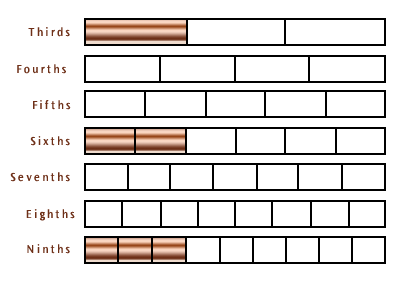Example 5: What are the missing numbers in this sequence?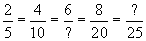The diagram below shows the pattern of these equivalent fractions. Can you fill in the missing numbers and then find the next equivalent fraction in the sequence?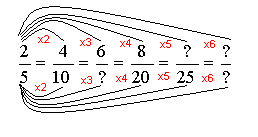To find each equivalent fraction in this sequence we have multiplied both the numerator and denominator of the original fraction, 2/5, by the same number for each fraction.

Example 6: What are the missing numbers?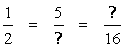In this example there is no obvious pattern to follow, but we can still work out the equivalent fractions using the same method. The diagram below shows the equivalent fractions.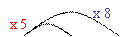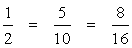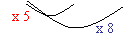To find each equivalent fraction in this example we have once again multiplied both the numerator and the denominator of the original fraction, 1/2, by the same number for each fraction.

Can you find 2 more greater equivalent fractions for one half?

 Using fractions everyday Our real-life use of fractions requires us to be able to relate commonly used fractions such as 1/2, 3/4, 2/3 to many of their equivalent fractions. For example, If there are 78 Year 7 students at our school and 34 ride their bikes to school regularly, then 34/78 students ride their bikes. 34/78 students is equivalent to 1/2 the Year 7 students. In many everyday situations we are only interested in approximations and will often approximate fractions to their common equivalents.

The above results suggest that for any fraction a/b,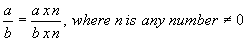Click here to see the explanation of this result at Justification for a way to find equivalent fractions.

Comparing fractions

Example 7: Which of these fractions is the largest, 1/9, 7/8, 1/2?

We can find the answer to this problem by roughly visualising the size of each of these fractions.

 Question Thinking Which of these fractions is the largest: 1/9, 7/8, 1/2? For ninths, one is broken into nine equal parts. For eighths, one is broken into 8 equal parts. So one eighth is more than one ninth. 7 eighths is nearly all the eighths in one, so it is close to one, whereas one half is midway between zero and one. SO, the largest is 7 eighths and the smallest is 1 ninth.

In some cases, we can visualise common fractions and can recognise the difference between fractions without the need to use more formal mathematical procedures to compare them.

Example 8: Which fraction is largest, 2/3, 3/4 or 5/6?

It is possible to mentally picture each of these fractions using the rectangle and estimate which is largest, but another way is to change each fraction so that they all have the same denominator. We call fractions which have the same denominator like fractions which means they have a common denominator.

The next section explains methods we can use for Finding common denominators.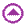Finding common denominators

In order to compare the fractions 2/3, 3/4 and 5/6 we can find an equivalent fraction for each one all with the same denominator. This will make the comparison easy. To do this, we simply list the sequence of equivalent fractions for each number:

2/3 = 4/6 = 6/9 = 8/12 = 10/15 = 12/18 = 14/21 = 16/24 = 18/27 = ..

3/4 = 6/8 = 9/12 = 12/16 = 15/20 = 18/24 = 21/28 = 24/32 = ..

5/6 = 10/12 = 15/18 = 20/24 = 25/30 = 30/36 = 35/42 = ..

Twelve occurs as a denominator on all three lists. Thus 2/3, 3/4 and 5/6 can be rewritten as 8/12, 9/12, 10/12. Clearly, it can be seen that 10/12 represents the largest fraction. Hence 5/6 (which is equivalent to 10/12) is larger than 2/3 or 3/4.

Each fraction has a denominator of 12. Twelve is a common denominator for these three fractions.

The list shows us that 24 is another common denominator for these three fractions, and there are others - can you find them? (Hint: continue the lists and look for a pattern).

Because 12 is the lowest of all the common denominators for these three fractions, it is called the LOWEST COMMON DENOMINATOR. When we are comparing fractions we usually try to use the lowest common denominator so that we are working with smaller numbers, but this is not essential. Any common denominator can be used.

Another way of finding a common denominator is to multiply all the denominators together - can you explain why this works and can you find out when this method gives the lowest common denominator?

Finding the lowest common denominator

To find the lowest common denominator for the fractions of 2/3, 3/4 and 5/6 we could have used either of these methods:

 Working: Thinking 3 x 4 = 12, 6 x 2 = 12 look at each denominator and recognise that 12 is a multiple of 3,4 and 6 - in fact it is the lowest common multiple 3,6,9,12,15,18,21,24.. 4,8,12,16,20,24.. 6,12,24.. list the multiples for each denominator find the common multiples of the denominators (12 and 24) choose the smallest common multiple (12)

Lowest common denominators can also be systematically found by considering the prime factors of the denominators. This is the best method for large numbers, but the methods above are easy to carry out in most cases and quick to teach.Using multiplication of fractions to find equivalent fractions

If you are familiar with how to multiply fractions you can use this method to find equivalent fractions.

In order to find equivalent fractions you need to understand that the value of a fraction is unaltered when it is multiplied by one. One can be written as any number divided by itself (1 = n/n).

For example, 1 is the same as 2/2, 5/5 or 89/89, so we can multiply 2/3 by any of these fractions without changing its value.

2/3 x 2/2 = 4/6 = 2/3

2/3 x 5/5 = 10/15 = 2/3

2/3 x 89/89 = 178/267 = 2/3

Example 9:

How do we change 1/2 into the equivalent fractions 3/6 or 5/10?

 Question Working Thinking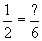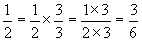can multiply the fraction by 1 without changing its value need to multiply 1/2 by 1 where 1 is named in a way to give a denominator of 6 2 x 3 = 6, so use 3/3 as the name for 1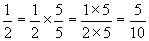can multiply the fraction by 1 without changing its value need to multiply 1/2 by 1 where 1 is named in a way to give a denominator of 10 2 x 5 = 10, so use 5/5 as the name for 1Justification for a way to find equivalent fractions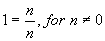We know: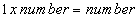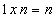We know: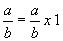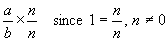Hence: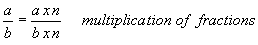Quick quiz

 1. Find 2 fractions which are equivalent to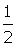2. Find 2 fractions which are equivalent to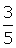3. Fill in the boxes with the missing equivalent fractions in the following sequences a)=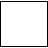==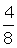===b)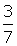=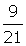===4. Complete: a)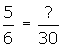b)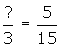c)5. Use equivalent fractions to find a common denominator (try to find the lowest common denominator) and arrange the following fractions in order from smallest to largest. a)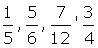b)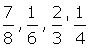c)